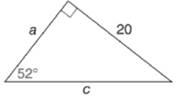Chapter 11.3, Problem 18EElementary Geometry For College St...

7th Edition
Alexander + 2 others
ISBN: 9781337614085

Solutions

Chapter
SectionElementary Geometry For College St...

7th Edition
Alexander + 2 others
ISBN: 9781337614085
Textbook Problem

In Exercises 15 to 20, use the sine, cosine, or tangent ratio to find the lengths of the indicated sides to the nearest tenth of a unit.To determine

To find:

The lengths of the indicated sides to the nearest tenth of a unit using the sine, cosine or tangent ratio.

Explanation

Given:

The triangle with the indicated sides is shown below.

Definition used:

The trigonometric ratios:

Calculation:

Let α=52.

From the figure we see that opposite=20,adjacent=a,hypotenuse=c.

We need to find the values of a, c.

We have sinα=oppositehypotenuse

sin52=20c

tan52=20a

Let us use Ti-83 calculator to find the value of sin52,tan52.

Press ON in Ti-83 calculator.

Since we need to find the value of degree, we need to change the mode to degrees.

Press MODE button and use arrow keys to select the “Degree” mode.

Let us find the values, correct to four decimal places.

Since we need the values of sin52,tan52 correct to four decimal places, in MODE, using arrow keys choose the value of “Float” as 4

Still sussing out bartleby?

Check out a sample textbook solution.

See a sample solution

The Solution to Your Study Problems

Bartleby provides explanations to thousands of textbook problems written by our experts, many with advanced degrees!

Get Started

10003/2

Applied Calculus for the Managerial, Life, and Social Sciences: A Brief Approach

Fill in each blank: 88oz=lb

Elementary Technical Mathematics

Convert the expressions in Exercises 31-36 to positive exponent form. 12x4

Finite Mathematics and Applied Calculus (MindTap Course List)

Evaluate . e + 1

Study Guide for Stewart's Single Variable Calculus: Early Transcendentals, 8th

True or False: converges.

Study Guide for Stewart's Multivariable Calculus, 8th